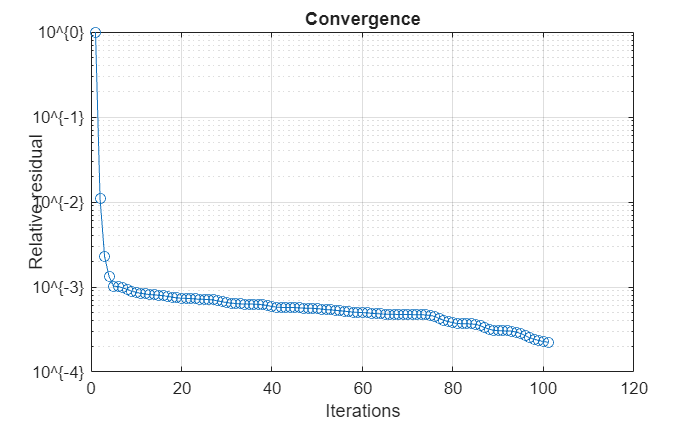# solver

Access FMM solver for electromagnetic analysis

Since R2021b

## Description

Use the `solver` object to access the fast multipole method (FMM) solver settings in large antenna structures, such as egg crate, installed antenna, and curved reflectors, for electromagnetic (EM) analysis.

## Creation

### Syntax

``s = solver(obj)``

### Description

example

``` `s = solver(obj)` returns the solver used in the antenna defined in `obj` for EM analysis. For more information on EM analysis, see Antenna and Array Analysis. After you create a `solver` object, you can modify the object properties using dot notation. NoteYou must set the `SolverType` property to `'FMM'` in the egg crate, installed antenna, or curved reflectors antenna objects to access the `solver` object. ```

### Input Arguments

expand all

Large antenna, specified as one of the following:

Antenna NameAntenna Objects
Egg crate`eggCrate`
Installed antenna`installedAntenna`
Curved reflectors

Example: `s = solver(obj)`

## Properties

expand all

Maximum number of iterations needed by the FMM solver to achieve convergence, specified as a positive scalar. During EM analysis, if convergence is achieved with fewer iterations than the maximum number of iterations specified using this property, the FMM solver terminates the EM analysis and displays the EM analysis solutions.

Example: `obj.Iterations = 150;`

Residual error allowed in EM solution, specified as a positive scalar.

Example: `obj.RelativeResidual = 1.5e-3;`

FMM solver precision, specified as a positive scalar.

Example: `obj.Precision = 2.0000e-5;`

## Object Functions

 `convergence` Calculate and plot convergence of FMM solver

## Examples

collapse all

Design a default parabolic reflector antenna.

`m = reflectorParabolic;`

Set the solver type of the parabolic reflector antenna to FMM.

`m.SolverType = 'FMM';`

Calculate the impedance of the parabolic reflector antenna at 10 GHz.

`Z = impedance(m,10e9);`

Access the FMM solver and set the relative residual to 1e-3.

```s = solver(m); s.RelativeResidual = 1e-3;```

Calculate and plot the convergence of the FMM solver for the parabolic reflector antenna.

`convergence(s)`## Version History

Introduced in R2021b